# RBSE Maths Class 9 Chapter 6: Important Questions and Solutions

RBSE Maths Chapter 6 -Rectilinear Figures Class 9 Important questions and solutions are available at BYJU’S. The important questions and solutions of Chapter 6 are given here by considering the new pattern of RBSE. Also, RBSE Class 9 solutions are useful for the students to clear their exams with maximum marks.

Chapter 6 of the RBSE Class 9 Maths will help the students to solve problems related to plane figures like triangles. Different properties of plane figures are involved in this chapter, which helps the students in solving problems related to missing angles. After practicing all these important questions, students will be able to understand how to apply the properties of plane figures in different scenarios.

### RBSE Maths Chapter 6: Exercise 6.1 Textbook Important Questions and Solutions

Question 1: In the figure, find all the angles of ∆ABC.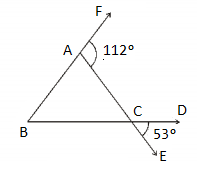Solution:

Given,

∠DCE = 53°

∠DCE = ∠ACB = 53° (vertically opposite angles)

In triangle ABC,

∠ABC + ∠ACB = ∠CAF (by exterior angle property)

∠ABC + 53° = 112°

∠ABC = 112° – 53° = 59°

Again, in triangle ABC,

By angle sum property of a triangle,

∠BAC + ∠ABC + ∠BCA = 180°

∠BAC + 59° + 53° = 180°

∠BAC = 180° – 59° – 53° = 68°

Therefore, ∠BAC = 68°, ∠ABC = 59°, ∠ACB = 53°

Question 2: In the figure, ∆ABC is an equilateral triangle. Find the values of ∠x, ∠y and ∠z.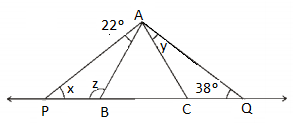Solution:

Given that, ∆ABC is an equilateral triangle.

Therefore, AB = BC = CA

And ∠ABC = ∠BAC = ∠ACB = 60°

∠ABC = ∠BAP + ∠APB (exterior angle property)

60° = 22° + ∠x

∠x = 60° – 22° = 38°

In triangle APB,

By the angle sum property,

∠x + ∠z + 22° = 180°

38° + ∠z + 22° = 180°

∠z = 180° – 38° – 22° = 120°

Again, ∠ACB = 38° + y (by exterior angle property)

60° = ∠y + 38°

∠y = 60° – 38° = 22°

Thus, ∠x = 38°, ∠y = 22° and ∠z = 120°.

Question 3: In the figure, sides AB and AC of ∆ABC are produced to E and D, respectively. If angle bisectors BO and CO of ∠CBE and ∠BCD meet each other at point O, then prove that: ∠BOC = 90° – ∠x/2.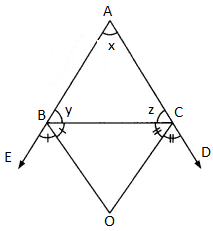Solution:

Given that, BO is the bisector of ∠CBE.

Therefore, ∠CBO = (1/2) ∠CBE

∠CBO = (½)(180° – y)

=90° – y/2 ….(i)

Similarly, ray OC is the bisector of angle BCD.

Therefore, ∠BCO = (½) ∠BCD

= (½)(180° – z)

= 90° – z/2 ….(ii)

In triangle BOC,

∠BOC + ∠BCO + ∠CBO = 180° ….(iiI) (by angle sum property)

Substituting (i) and (ii) in (iii),

∠BOC + 90° – z/2 + 90° – y/2 = 180°

∠BOC = z/2 + y/2

= (½) (y + z)

But, x + y + z = 180 (by angle sum property)

y + z = 180° – x

∠BOC = (½)(180° – x)

= 90° – x/2

Hence proved.

Question 4: In the figure, if AB ∥ DE, ∠BAC = 35 and ∠CDE = 53, then find the value of ∠DCE.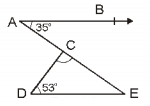Solution:

Given,

AB ∥ DE

Thus, ∠BAC = ∠DEC (alternate Interior Angles)

∠BAC = 35° (Given)

Therefore, ∠DEC = 35°

In ∆DCE,

By angle sum property of a triangle,

∠DEC + ∠CDE + ∠DCE = 180°

35° + 53° + ∠DCE = 180°

∠DCE = 180°- 35° – 53° = 92°

Question 5: In the figure, if lines PQ and RS intersect each other at point T, such that ∠PRT = 40°, ∠RPT = 95° and ∠TSQ = 75°, then find ∠SQT.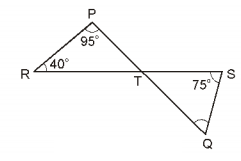Solution:

Given,

∠PRT = 40°, ∠RPT = 95° and ∠TSQ = 75°

In ∆PRT,

By the angle sum property of a triangle,

∠PRT + ∠RPT + ∠RTP = 180°

40° + 95° + ∠RTP = 180°

∠RTP = 180° – 40° – 95° = 45°

∠RTP = ∠QTS (vertically opposite angles)

Therefore, ∠QTS = 45°

In ∆STQ,

By the angle sum property of a triangle,

∠SQT + ∠TSQ + ∠QTS = 180°

∠SQT + 75° + 45° = 180°

∠SQT = 180° – 75° – 45° = 60°

Hence, ∠SQT = 60°

Question 6: In the figure, sides QP and RQ of a triangle PQR are produced upto S and T, respectively. If ∠SPR = 135° and ∠PQT = 110°, then find ∠PRQ.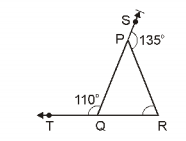Solution:

Given,

∠SPR = 135° and ∠PQT = 110°

From the given figure,

∠SPR +∠QPR = 180° (by linear pair axiom)

135° +∠QPR = 180°

∠QPR = 180° – 135°

∠QPR = 45°

∠PQT +∠PQR = 180° (by linear pair axiom)

⇒ 110° + ∠PQR = 180°

⇒ ∠PQR = 70°

Now, in triangle PQR,

By the angle sum property,

∠PQR +∠QPR + ∠PRQ = 180°

⇒ 70° + 45° + ∠PRQ = 180°

⇒ 115° + ∠PRQ = 180°

⇒ ∠PRQ = 180° – 115° = 65°

Question 7: In the figure, if PQ ⊥ PS, PQ ∥ SR, ∠SQR = 28 and ∠QRT = 65, then find the values of x and y.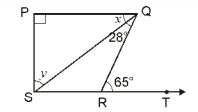Solution:

Given,

PQ∥SR.

We know that alternate interior angles are equal.

Therefore, ∠QRT=∠PQR

⇒ 65° = x + 28°

⇒ x = 65° − 28° = 37°

In ∆PQS,

By the angle sum property,

x + y + ∠SPQ = 180°

⇒ 37° + y + 90° = 180° ( given ∠SPQ = 90°)

⇒ y = 180° – 37° – 90° = 53°

Therefore, x = 37° and y = 53°

Question 8: In the figure, side QR of ∆PQR is produced to point S. If the bisectors of ∠PQR and ∠PRS meets at point T, then prove that: ∠QTR = (1/2) ∠QPR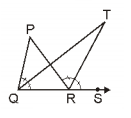Solution:

Given that bisectors of ∠PQR and ∠PRS meet at point T.

We know that the exterior angle of a triangle is equal to the sum of the two interior angles.

∠TRS = ∠TQR +∠QTR

⇒∠QTR = ∠TRS – ∠TQR ….(i)

∠SRP = ∠QPR + ∠PQR

⇒ 2∠TRS = ∠QPR + 2∠TQR (since TR is a bisector of ∠SRP and QT is a bisector of ∠PQR)

⇒∠QPR = 2∠TRS – 2∠TQR

⇒∠QPR = 2(∠TRS – ∠TQR)

⇒ 1/2∠QPR = ∠TRS – ∠TQR …. (ii)

From (i) and (ii),

∠QTR = 1/2∠QPR

Hence proved.

Question 9: In triangle ABC, A is right angle. L is any point on BC such that AL ⊥ BC. Prove that: ∠BAL = ∠ACB.

Solution:

Let us draw the triangle ABC with the right angle at A.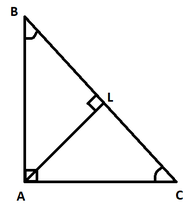In triangle ALB,

By the angle sum property,

∠ALB + ∠BAL + ∠ABL = 180°

90° + ∠BAL + ∠ABC = 180°

∠BAL + ∠ABC = 180° – 90°

∠BAL + ∠ABC = 90° ….(i)

Again in triangle ABC,

By the angle sum property

∠BAC + ∠ACB + ∠ABC = 180°

90° + ∠ACB + ∠ABC = 180°

∠ACB + ∠ABC = 180° – 90°

∠ACB + ∠ABC = 90° ….(ii)

From (i) and (ii),

∠BAL + ∠ABC = ∠ACB + ∠ABC

∠BAL = ∠ACB

Hence proved.

Question 10: The angles of a triangle are in the ratio 2 : 3 : 4. Find all the angles of the triangle.

Solution:

Given,

Ratio of all the three angles of a triangle = 2 : 3 : 4

Let 2x, 3x and 4x be the angles of the triangle.

By the angle sum property of a triangle,

2x + 3x + 4x = 180°

9x = 180°

x = 180°/9

x = 20°

Thus, 2x = 2(20°) = 40°

3x = 3(20°) = 60°

4x = 4(20°) = 80°

Therefore, the angles of the triangle are 40°, 60° and 80°.

### RBSE Maths Chapter 6: Exercise 6.2 Textbook Important Questions and Solutions

Question 11: If a regular polygon has 8 sides, then

(i) find the sum of its exterior angles.

(ii) find the measure of each exterior angle.

(iii) find the sum of its interior angles.

(iv) find the measure of each interior angle.

Solution:

(i) The sum of all exterior angles of the polygon = 360°

(ii) Measure of each exterior angle = 360°/8 = 45°

(iii) Sum of all the interior angles = (2n – 4) × 90°

= (2 × 8 – 4) × 90°

= (16 – 4) × 90°

= 12 × 90°

= 1080°

(iv) Measure of each interior angle = 1080°/8 = 135°

Question 12: If the sum of interior angles of a regular polygon is 2160°, then what will be the number of sides of the polygon? Find it.

Solution:

Given that, the sum of the interior angles of a polygon = 2160°

(2n – 4) × 90° = 2160°

2n – 4 = 2160°/90°

2n – 4 = 24

2n = 24 + 4 = 28

n = 28/2 = 14

Therefore, the number of sides of the polygon = 14

Question 13: Check whether a regular polygon is possible with each interior angle 137°.

Solution:

Given,

The measure of each interior angle = 137°

Thus, measure of each exterior angle = 180° – 137° = 43°

Let n be the number of sides of the polygon.

We know that the sum of all exterior angles = 360°

Therefore, n = 360/43 = 8.37209..

Which is not a whole number.

Hence, it is not possible for a polygon to have an interior angle measure 137°.

Question 14: Find ∠CED and ∠BDE in the following figure.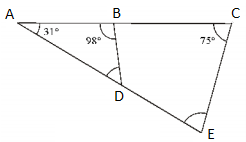Solution:

In triangle ABD,

By the angle sum property,

∠A + ∠B + ∠BDA = 180°

31° + 98° + ∠BDA = 180°

∠BDA = 180° – 98° – 31° = 51°

∠BDA and ∠BDE form a linear pair.

Thus, ∠BDE = 180° – ∠BDA

= 180° – 51°

= 129°

In triangle AEC,

By the angle sum property,

∠CEA = 180° – 31° – 75° = 74°

∠CEA = ∠CED (corresponding angles)

Therefore, ∠BDE = 129° and ∠CED = 74°

### RBSE Maths Chapter 6: Additional Important Questions and Solutions

Question 1: If two angles of a triangle are of measure 90° and 30°, then the third angle is:

(A) 90°

(B) 30°

(C) 60°

(D) 120°

Solution:

Given measures of two angles are 90° and 30°.

Let x be the third angle.

We know that the sum of all the three angles of a triangle is 180°.

90° + 30° + x = 180°

120° + x = 180°

x = 180° – 120° = 60°

Question 2: Three angles of triangle are in the ratio 2 : 3 : 4, then the measure of its greatest angle is:

(A) 80°

(B) 60°

(C) 40°

(D) 180°

Solution:

Given,

Ratio of all the three angles of the triangle = 2 : 3 : 4

Let 2x, 3x and 4x be the angles of the triangle.

By the angle sum property of a triangle,

2x + 3x + 4x = 180°

9x = 180°

x = 180°/9

x = 20°

Thus, 2x = 2(20°) = 40°

3x = 3(20°) = 60°

4x = 4(20°) = 80°

Hence, the measure of greatest angle = 80°

Question 3: Measure of each angle of an equilateral triangle is:

(A) 90°

(B) 30°

(C) 45°

(D) 60°

Solution:

We know that, in an equilateral triangle all the three angles are equal.

Therefore, each angle measures 60°

Question 4: Four angles of a quadrilateral are in the ratio 1 : 2 : 3 : 4, then the measure of its smallest angle is:

(A) 120°

(B) 36°

(C) 18°

(D) 10°

Solution:

Given, the ratio of four angles of a quadrilateral is 1 : 2 : 3 : 4.

Let x, 2x, 3x and 4x be the four angles of the quadrilateral.

Thus,

x + 2x + 3x + 4x = 360°

10x = 360°

x = 360°/10 = 36°

2x = 3(36°) = 72°

3x = 3(36°) = 108°

4x = 4(36°) = 144°

Therefore, the smallest angle is 36°.

Question 5: In the figure, side BC of triangle ABC is produced upto point D. If ∠A = 55° and ∠B = 60°, then the measure of ∠ACD is: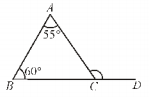(A) 120°

(B) 110°

(C) 115°

(D) 125°

Solution:

By the exterior angle property,

∠ACD = ∠A + ∠B

= 55° + 60°

= 115°

Question 6: Sum of all interior angles of a hexagon is:

(A) 720°

(B) 360°

(C) 540°

(D) 1080°

Solution:

We know that, the number of sides of a hexagon = n = 6

Sum of all interior angles = (2n – 4) × 90°

= (2 × 6 – 4) × 90°

= (12 – 4) × 90°

= 8 × 90°

= 720°

Question 7: The sum of exterior angles made by producing sides (in some order) of n-sided regular polygon:

(A) n-right angles

(B) 2n right angles

(C) (2n – 4) right angles

(D) 4 right angles

Solution:

We know that the sum of all exterior angles of any polygon is 360°.

That means, 4 right angles.

(4 × 90°) = 360°

Question 8: If one angle of a triangle is equal to the sum of other two angles, then the triangle is:

(A) Isosceles triangle

(B) Obtuse angled triangle

(C) Equilateral triangle

(D) Right angled triangle

Solution:

Let x, y, z be the angles of a triangle.

x = y + z (as per the given)

By the angle sum property,

x + y + z = 180°

x + x = 180°

2x = 180°

x = 180°/2 = 90°

Hence, the triangle is the right triangle.

Question 9: In a regular polygon. number of sides is n, then the measure of each interior angle is:

(A) 360°/n

(B) (2n – 4)/n right angles

(C) n right angles

(D) 2n right angles

Solution:

Sum of all interior angles = (2n – 4) × 90°

Measure of each interior angle = [(2n – 4)/n] × 90°

That means, (2n – 4)/n right angles

Question 10: The exterior angle of a triangle is 105° and the interior opposite angles are the same. Each of the equal angle is:

(A) 37 1/2°

(B) 52 1/2°

(C) 72 1/2°

(D) 75°

Solution:

Let x be the measure of each equal interior angle.

By the exterior angle property,

x + x = 105°

2x = 105°

x = 105° = 52 1/2°

Question 11: The angles of a triangle are in the ratio 5 : 3 : 7. This triangle is:

(A) Acute angled triangle

(B) Obtuse angled triangle

(C) Right angled triangle

(D) Isosceles triangle

Solution:

Given,

Ratio of all the three angles = 5 : 3 : 7

Let 5x, 3x and 7x be the angles of the triangle.

By the angle sum property of a triangle,

5x + 3x + 7x = 180°

15x = 180°

x = 180°/15

x = 12°

Thus, 5x = 5(12°) = 60°

3x = 3(12°) = 36°

7x = 7(12°) = 84°

Hence, the triangle is an acute angle triangle.

Question 12: If one angle of a triangle is 130°, then angle between the angular bisector of two angles of a triangle may be:

(A) 50°

(B) 65°

(C) 145°

(D) 155°

Solution:

Let x, y and z be the three angles of a triangle.

Let x = 130°

By the angle sum property of a triangle,

x + y + z = 180°

130° + y + z = 180°

y + z = 180° – 130° = 50°

(y + z)/2 = 50°/2 = 25°

Therefore, angle between the angular bisectors of two angles y and z = 180° – 25° = 155°

Question 13: From the figure, find ∠A.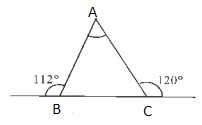Solution:

From the given figure,

∠ACB + 120° = 180° (by linear pair axiom)

∠ACB = 180° – 120° = 60°

By exterior angle property,

∠B = ∠A + ∠ACB

112° = ∠A + 60°

∠A = 112° – 60° = 52°

Question 14: In the figure, ∠B = 60° and ∠C = 40°. Find the measure of ∠A.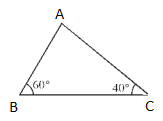Solution:

Given,

∠B = 60° and ∠C = 40°

In triangle ABC,

Sum of three angles = 180°

∠A + ∠B + ∠C = 180°

∠A + 60° + 40° = 180°

∠A = 180° – 100°

∠A = 80°

Question 15: In the figure, m ∥ QR, then find ∠QPR.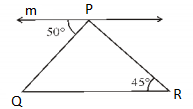Solution:

Given that,

m ∥ QR

Here, PR acts as a transversal.

Thus, ∠QPR = 180° – (50° + 45°)

= 180° – 95°

= 85°

Question 16: In the figure, find ∠A.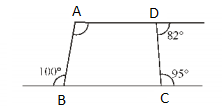Solution:

At the vertex B,

100° + ∠ABC = 180° (by linear pair axiom)

∠ABC = 180° – 100° = 80°

At the vertex C,

∠DCB + 95° = 180° (by linear pair axiom)

∠DCB = 180° – 95° = 85°

At the vertex D,

∠CDA + 82° = 180°

∠CDA = 180° – 82° = 98°

Sum of the all interior angles = 360°

∠A + ∠ABC + ∠DCB + ∠CDA = 360°

∠A + 80° + 85° + 98° = 360°

∠A + 263° = 360°

∠A = 360° – 263°

∠A = 97°

Question 17: Four interior angles of a pentagon are 40°, 75°, 125° and 135°, then find the measure of the fifth angle.

Solution:

Let x be the fifth angle of a pentagon.

We know that the sum of all interior angles of a pentagon = 540°

x + 40° + 75° + 125° + 135° = 540°

x + 375° = 540°

x = 540° – 375°

x = 165°

Therefore, the fifth angle of the pentagon = 165°

Question 18: If each exterior angle of a regular polygon is 45°, then find the number of its sides.

Solution:

If n is the number of sides, then the measure of each exterior angle of a polygon is 360°/n.

45° = 360°/n

n = 360°/45°

n = 8

Therefore, the number of sides of the polygon = 8

Question 19: The number of sides of a regular polygon is 12, then find the measure of each interior angle.

Solution:

If n is the number of sides, then the measure of each interior angle of a polygon = [(2n – 4)/n] × 90°

= [(2 × 12 – 4)/12] × 90°

= [(24 – 4)/12] × 90°

= (20/12) × 90°

= 150°

Hence, the measure of each interior angle is 150°.

Question 20: Sum of all interior angles of a polygon is 10 right angles. Find the number of sides.

Solution:

If n is the number of sides of a polygon, then the sum of interior angles = (2n – 4) × 90°

According to the given,

10 × 90° = (2n – 4) × 90°

2n – 4 = 10

2n = 10 + 4

2n = 14

n = 14/2 = 7

Therefore, the number of sides of the polygon = 7

Question 21: Check whether a polygon is possible if each of its interior angles is of measure 110°.

Solution:

Given,

The measure of each interior angle = 110°

Thus, measure of each exterior angle = 180° – 110° = 70°

Let n be the number of sides of the polygon.

We know that the sum of all exterior angles = 360°

Therefore, n = 360/70 = 5.14285..

Which is not a whole number.

Hence, it is not possible for a polygon to have an interior angle measure 110°.

Question 22: If in a ΔABC, ∠A + ∠B = ∠C, then find the greatest angle of ΔABC.

Solution:

Given that in the triangle ABC, ∠A + ∠B = ∠C.

∠A + ∠B + ∠C = 180° (by the angle sum property of a triangle)

∠C + ∠C = 180°

2∠C = 180°

∠C = 180°/2 = 90°

Therefore, the greatest angle of the triangle is the right angle, i.e. 90°.

Question 23: Find the sum of interior angles of an octagon.

Solution:

If n is the number of sides of a polygon, then the sum of interior angles = (2n – 4) × 90°

The number of sides of an octagon = 8

Therefore,

Sum of interior angles = (2 × 8 – 4) × 90°

= (16 – 4) × 90°

= 12 × 90°

= 1080°

Question 24: Find each interior angle of regular decagon.

Solution:

The number of sides of decágon = 10

If n is the number of sides, then the measure of each interior angle of a polygon = [(2n – 4)/n] × 90°

= [(2 × 10 – 4)/10] × 90°

= [(20 – 4)/10] × 90°

= (16/10) × 90°

= 144°

Hence, the measure of each interior angle is 144°.

Question 25: 110°, 130° and x° are the exterior angles obtained by producing the sides of a triangle in the same order. Find the value of x.

Solution:

We know that the sum of exterior angles of a polygon = 360°

110° + 130° + x = 360°

240° + x = 360°

x = 360° – 240°

x = 120°

Question 26: If a hexagon has one interior angle of measure 165° and the remaining each interior angle of measure x°, then find the remaining angles.

Solution:

We know that the sum of all interior angles of a hexagon = 720°

165° + x + x + x + x + x = 720°

165° + 5x = 720°

5x = 720° – 165°

5x = 555°

x = 555°/5 = 111°

Therefore, the measure of remaining angles = 111°

Question 27: In the figure, AB ∥ DC, find ∠x, ∠y and ∠z.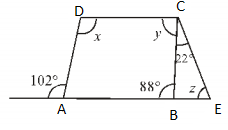Solution:

Given that,

AB ∥ DC

88° + ∠y = 180° (by the interior angle property)

∠y = 180° – 88°

∠y = 92°

In triangle BCE,

By the exterior angle property,

22° + ∠z = 88°

∠z = 88° – 22°

∠z = 66°

By the linear pair axiom,

102 + ∠DAB = 180

∠DAB = 180 – 102 = 78

Again by the interior angle property,

x + ∠DAB = 180°

x + 78° = 180°

x = 180° – 78°

∠x = 102°

Hence, ∠x = 102°, ∠y = 92°, and ∠z = 66°.

Question 28: In the below figure, find the value of ∠x and ∠y, where ∠x – ∠y = 10°.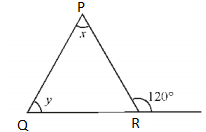Solution:

Given,

∠x – ∠y = 10° ….(i)

In triangle PQR,

By the exterior angle property,

∠x + ∠y = 120° ….(ii)

∠x – ∠y + ∠x + ∠y = 10° + 120°

2∠x = 130°

∠x = 130°/2

∠x = 65°

Substituting ∠x = 65° in equation (i),

65° – ∠y = 10°

∠y = 65° – 10°

∠y = 55°

Therefore, ∠x = 65° and ∠y = 55°

Question 29: In a polygon, two angles are of 90° each, remaining each angle is of measure 150°. Find the number of sides of this polygon.

Solution:

Let n be the number of sides of the polygon.

According to the given,

2 × 90° + (n – 2) × 150° = (2n – 4) × 90°

180° + 150°n – 300° = 180°n – 360

180°n – 150°n = 180° – 300° + 360°

30°n = 240°

n = 240°/30°

n = 8

Therefore, the number of sides of the polygon = 8

Question 30: In the figure, prove that: ∠x + ∠y = ∠A + ∠C.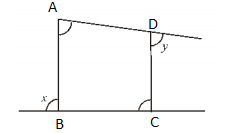Solution:

Let us divide the given quadrilateral into two triangles as shown below.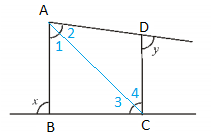In triangle ABC,

By the exterior angle property:

∠x = ∠1 + ∠3 ….(i)

∠y = ∠2 + ∠4 ….(ii)

∠x + ∠y = ∠1 + ∠3 + ∠2 + ∠4

∠x + ∠y = (∠1 + ∠2) + (∠3 + ∠4)

∠x + ∠y = ∠A + ∠C

Hence proved.

Question 31: In the figure, lines BO and CO are the bisectors of ∠B and ∠C. Find angle x.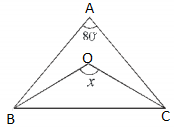Solution:

Given that, lines BO and CO are the bisectors of ∠B and ∠C.

Also, the sum of all three angles of a triangle is 180°.

Therefore,

∠BOC = 180° – (∠OBC + ∠OCB)

= 180 – (½) × 100°

= 180° – 50°

= 130°

Hence, the value of x = 130°

Question 32: In the figure, ∠Q > ∠R, PA is the bisector of QPR and PM ⊥ QR. Prove that: ∠APM = (1/2) (∠Q – ∠R)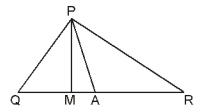Solution:

Given,

In ΔPQR, ∠ Q > ∠ R.

Also, PA is the bisector of ∠ QPR and PM ⊥ QR.

Since PA is the bisector of ∠P,

∠APQ = (1/2) ∠P ….(i)

In right triangle PMQ,

∠Q + ∠MPQ = 90°

⇒ ∠MPQ= 90°-∠Q ….(ii)

From the figure,

∴∠APM = ∠APQ – ∠MPQ

= 1/2 ∠P – (90° – ∠Q) [from (i) and (ii)]

= 1/2∠P – 90° + ∠Q

= 1/2∠P – 1/2(∠P + ∠R + ∠Q ) +∠Q

[since 90° = 1/2(∠P + ∠R + ∠Q)]

= 1/2(∠Q -∠R)

Hence proved.